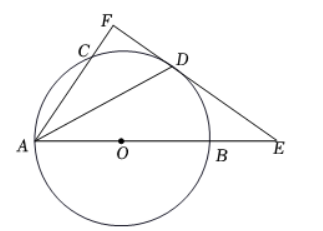(1)求证: $A F \perp E F$ ；
(2) 若 $C F=1 ， A C=2 ， A B=4$ ，求 $B E$ 的长.【答案】 （1）证明：连接 $OD$\begin{aligned} & \because A D \text { 平分 } \angle C A B ， \\ & \therefore \angle C A D=\angle O A D ， \\ & \because O A=O D ， \\ & \therefore \angle O A D=\angle O D A ， \\ & \therefore \angle C A D=\angle O D A ， \\ & \therefore O D \| A F ， \\ & \because E F \text { 为 } \odot O \text { 的切线， } \\ & \therefore O D \perp E F ， \\ & \therefore A F \perp E F . \end{aligned}

(2) 解: 由 (1) 得: $O D \| A F$ ，
\begin{aligned} & \therefore \triangle O D E \sim \triangle A F E, \\ & \because A C=2, C F=1, \\ & \therefore A F=3, \\ & \because A B=4 ， \\ & \therefore O D=2, O B=2, \\ & \therefore O E: A E=O D: A F ， \end{aligned}

\begin{aligned} & \therefore O E=O B+B E=2+x, \\ & \therefore \frac{2+x}{4+x}=\frac{2}{3}, \end{aligned}